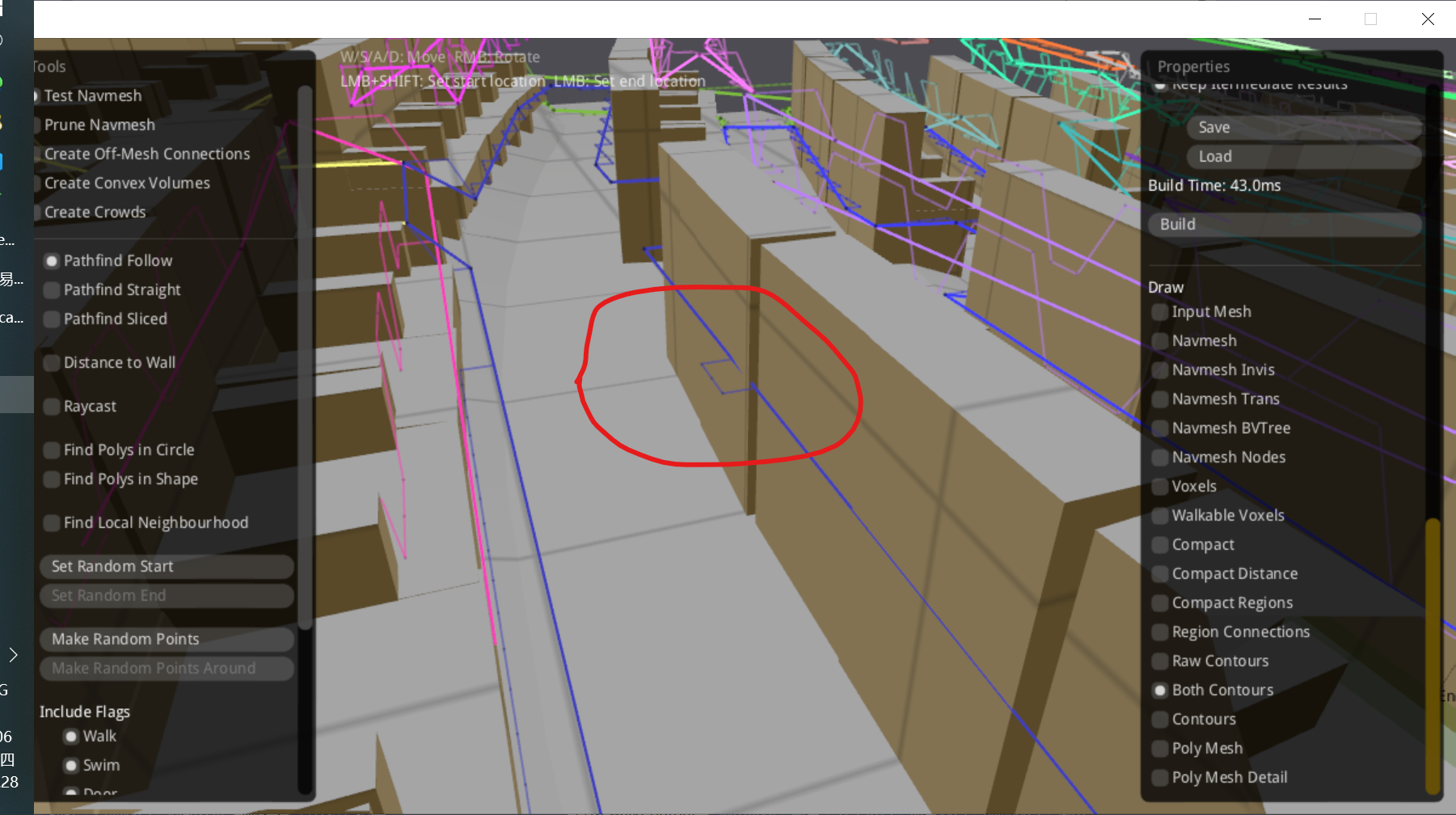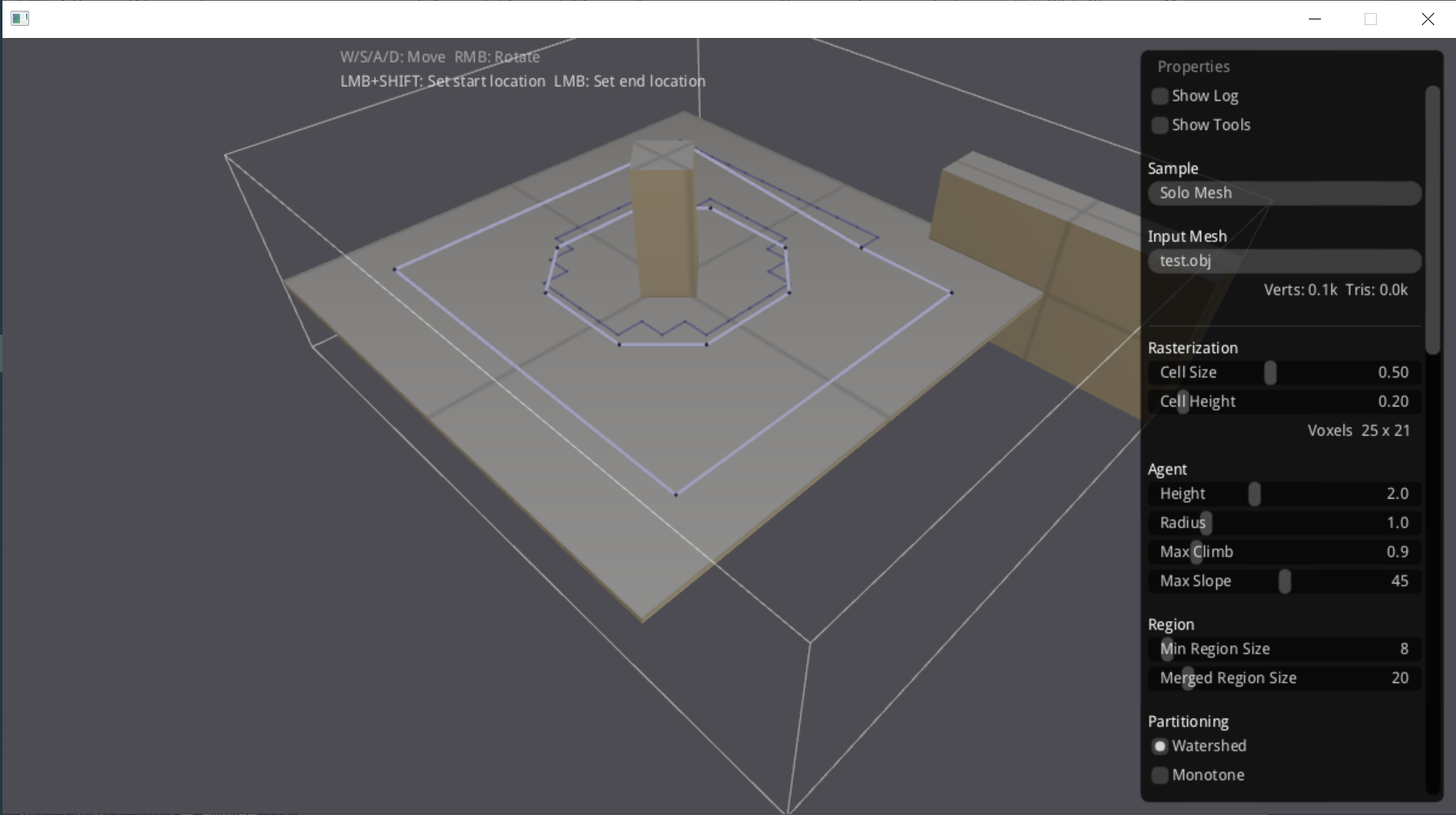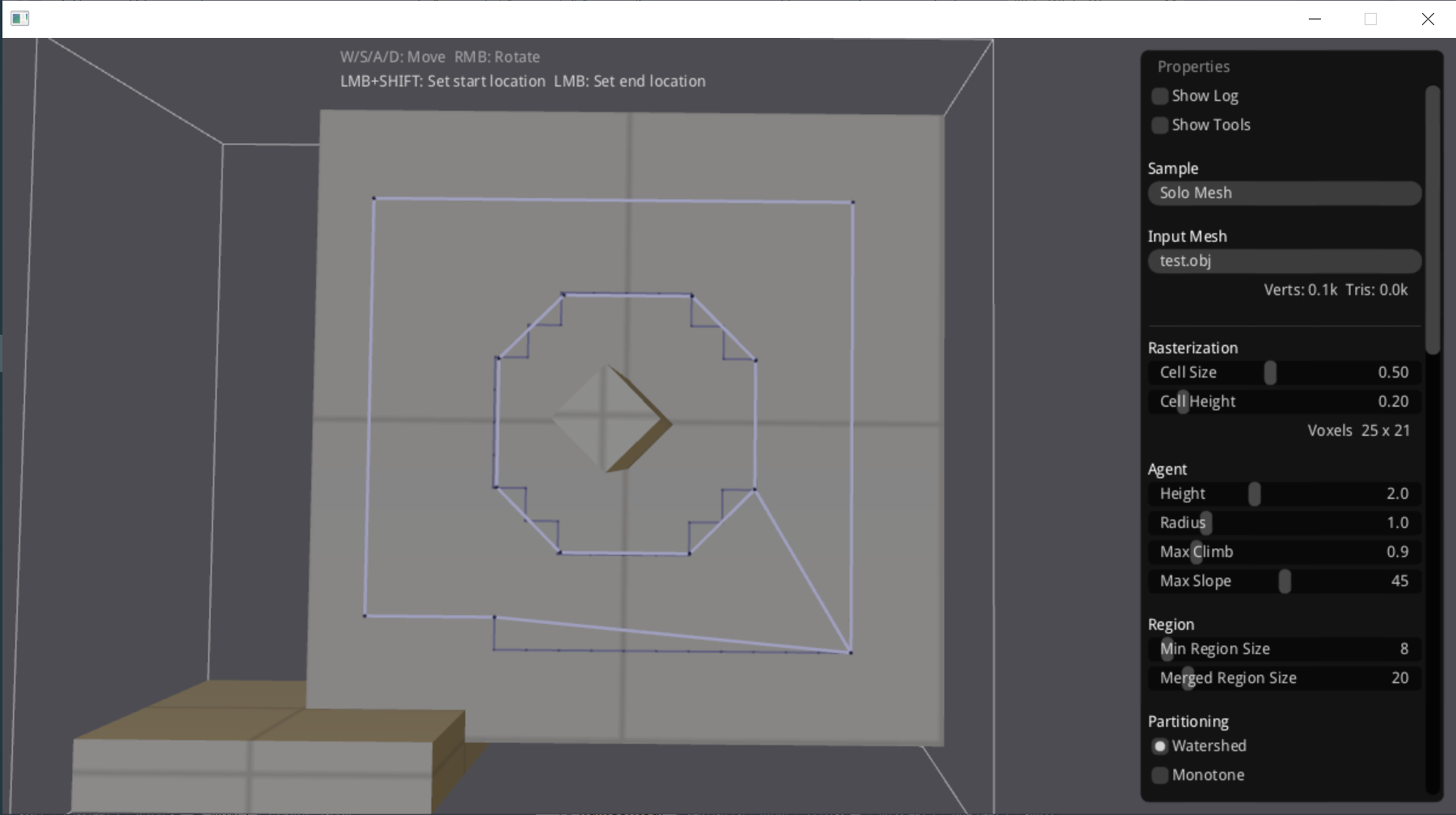# 金庆的专栏

C++博客 :: 首页 :: 新随笔 :: 联系 :: 聚合:: 管理 ::418 随笔 :: 0 文章 :: 454 评论 :: 0 Trackbacks

(Jin Qing's Column, Apr., 2022)

Using navmesh generated by recastnavigation, we found the agent will collide into the wall when it is walking along the navmesh path.

After some inspection, we found the reason is that at the collision position, the position on the path is simply too close to the wall, which is less than the agent radius.

If the agent radius is zero, the navmesh contour may inside the wall.Displaying the raw contour and contour, we can see the raw contour which is jigsaw shaped is right, but the simplied contour uses a straight line to replace the jigsaw edge, and causes the error.The parameter "Max Edge Error" controls how the contour is simplified, which default is 1.3, which means a vertex can deviate from the final contour in the range of 1.3 times of cell size.

We hope the error is on only one side of the contour instead of on both sides. In other words, the contour should within the raw contour.

walkContour() finds a raw contour which is a polygon. simplifyContour() simplifies the raw contour resulting a simpler polygon.

The steps of simplifyContour():

1. Find lower-left and upper-right vertices of the contour to form the initial simplified polygon.
2. For each edge of the simplified polygon
1. For each point between 2 points of the edge
1. Find the point that is the most distant from the edge
2. If the distance is larger than the max error, add the point to the simplified polygon
3. Split too long edges.

We make these modifications:

• The distance is signed
• If the point is outside the walkable area, just make sure it is less than the max error
• If the point is inside the walkable area
• Add it to the simplified polygon to make sure the final contour is within the raw area

There are 2 kinds of contour:

• Outline of a walkable area
• Hole of a walkable area

We should decide the kind of the contour before simplifyContour(). If the start cell (x, y) is in the raw contour polygon, then it is an outline not a hole contour. Because (x, y) may be one of the vetex of the polygon, we use (x + 0.5, y + 0.5) instead, which is the center of the cell.

The result will be like this:Compare with the orignal contour:posted on 2022-04-28 13:34 金庆 阅读(27) 评论(0)  编辑 收藏 引用